# Selina Solutions Concise Maths Class 7 Chapter 6: Ratio and Proportion (Including Sharing in a Ratio)

Selina Solutions Concise Maths Class 7 Chapter 6 Ratio and Proportion (Including Sharing in a Ratio) has answers to exercise wise problems in a comprehensive manner. Students who aspire to perform well in the annual exam are advised to solve problems using the solutions PDF. The main aim of creating solutions is to help students clear their doubts and improve conceptual knowledge. Selina Solutions Concise Maths Class 7 Chapter 6 Ratio and Proportion (Including Sharing in a Ratio), free PDF links are available below.

Chapter 6 provides students with the knowledge of ratio, conversion of ratio, proportion and continued proportion. The PDF of solutions is made available both chapter wise and exercise wise to help students ace the examination.

## Selina Solutions Concise Maths Class 7 Chapter 6: Ratio and Proportion (Including Sharing in a Ratio) Download PDF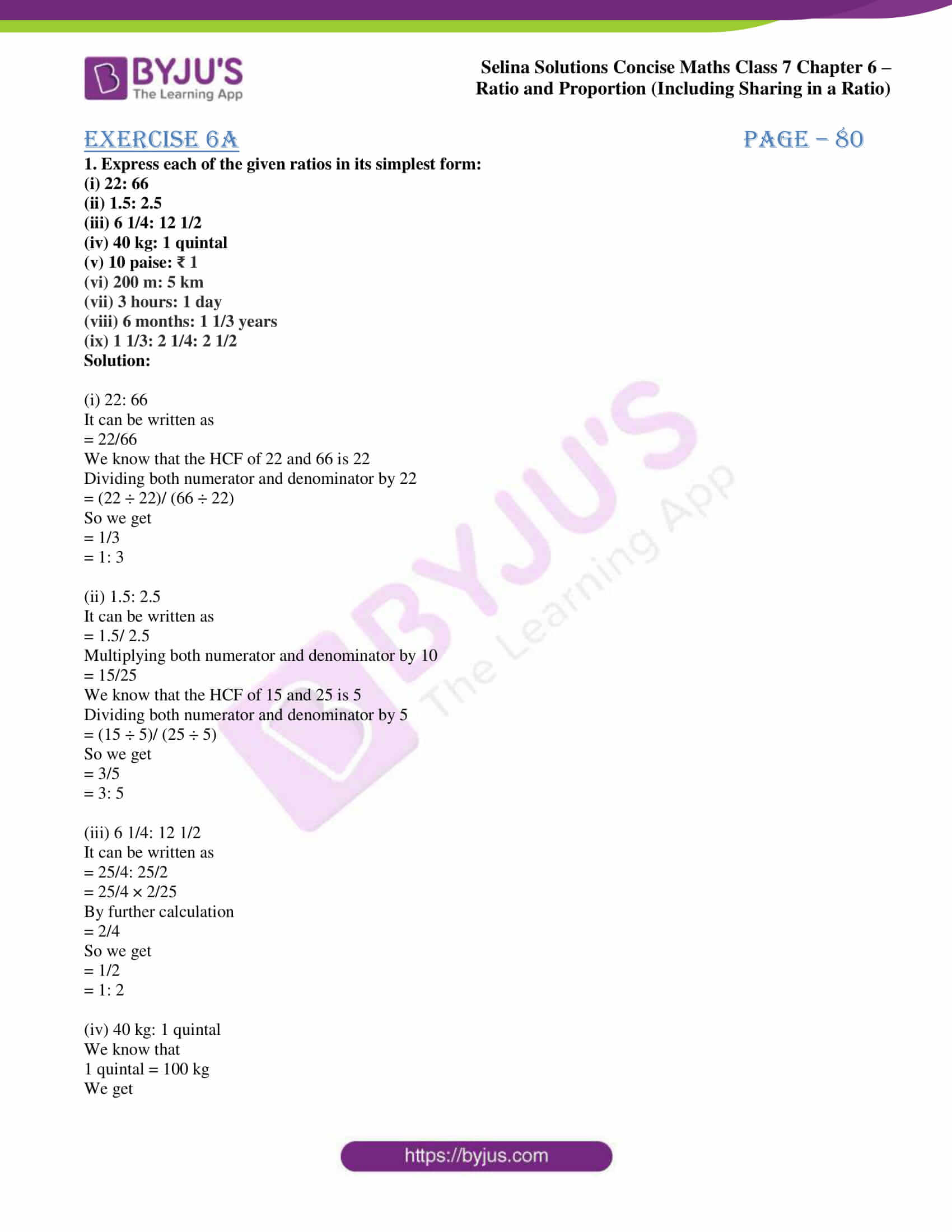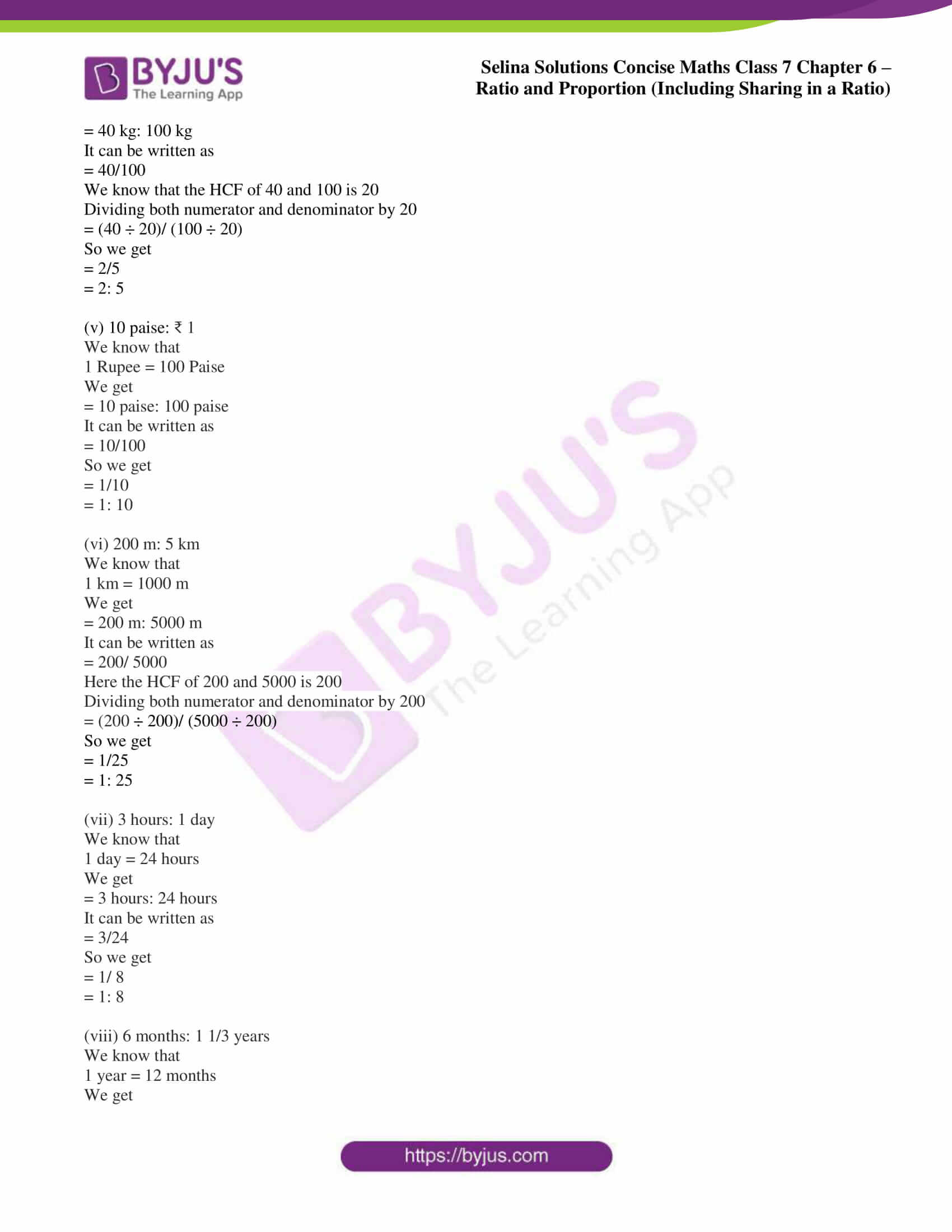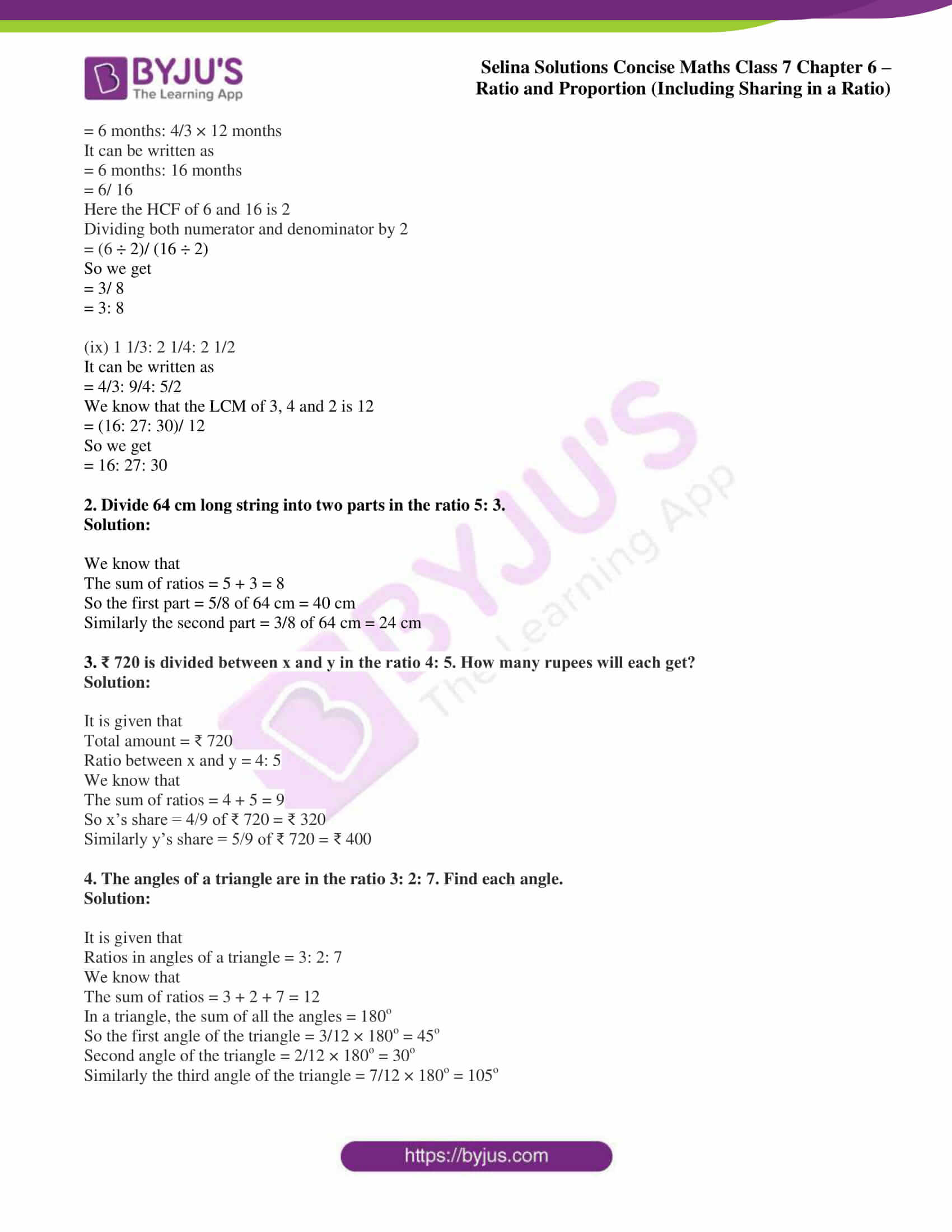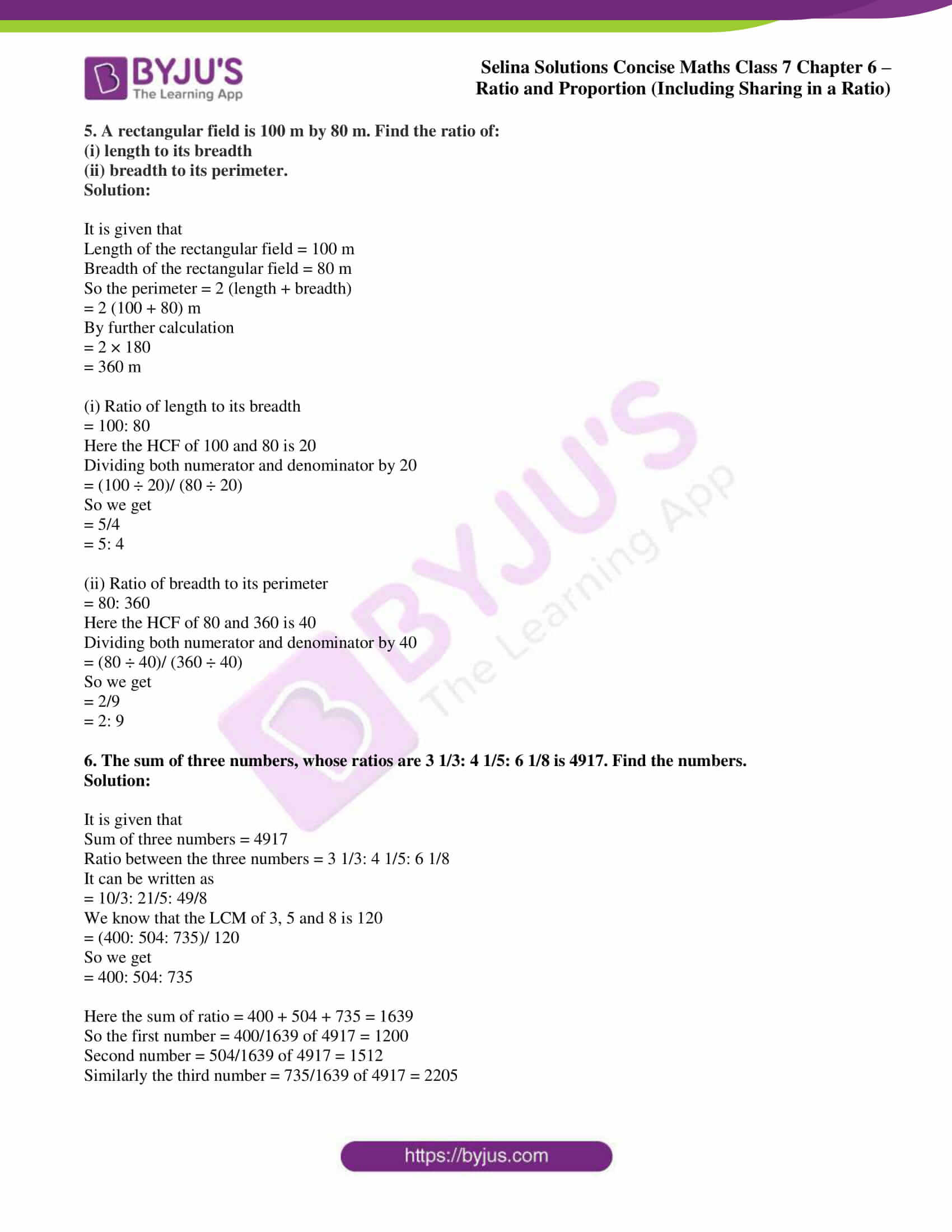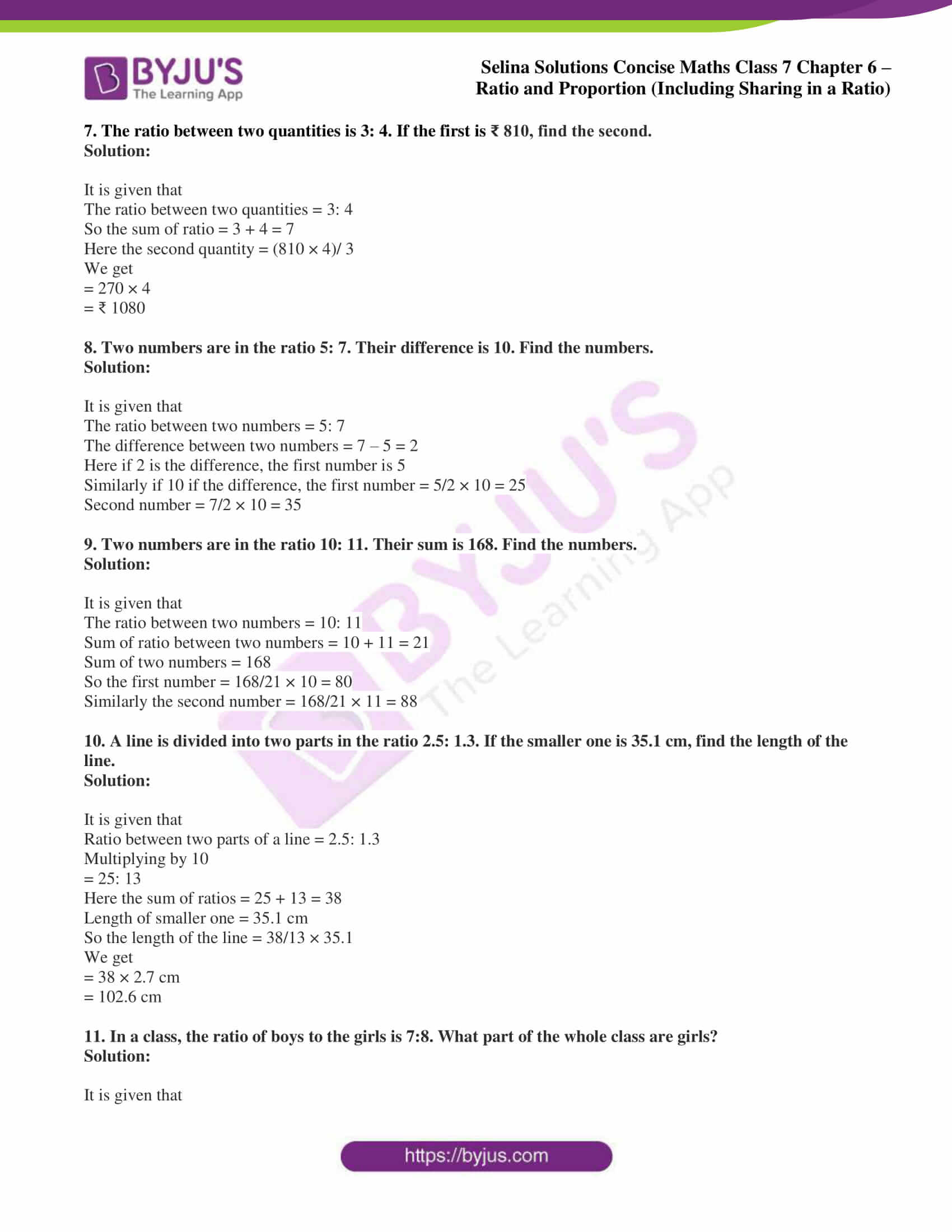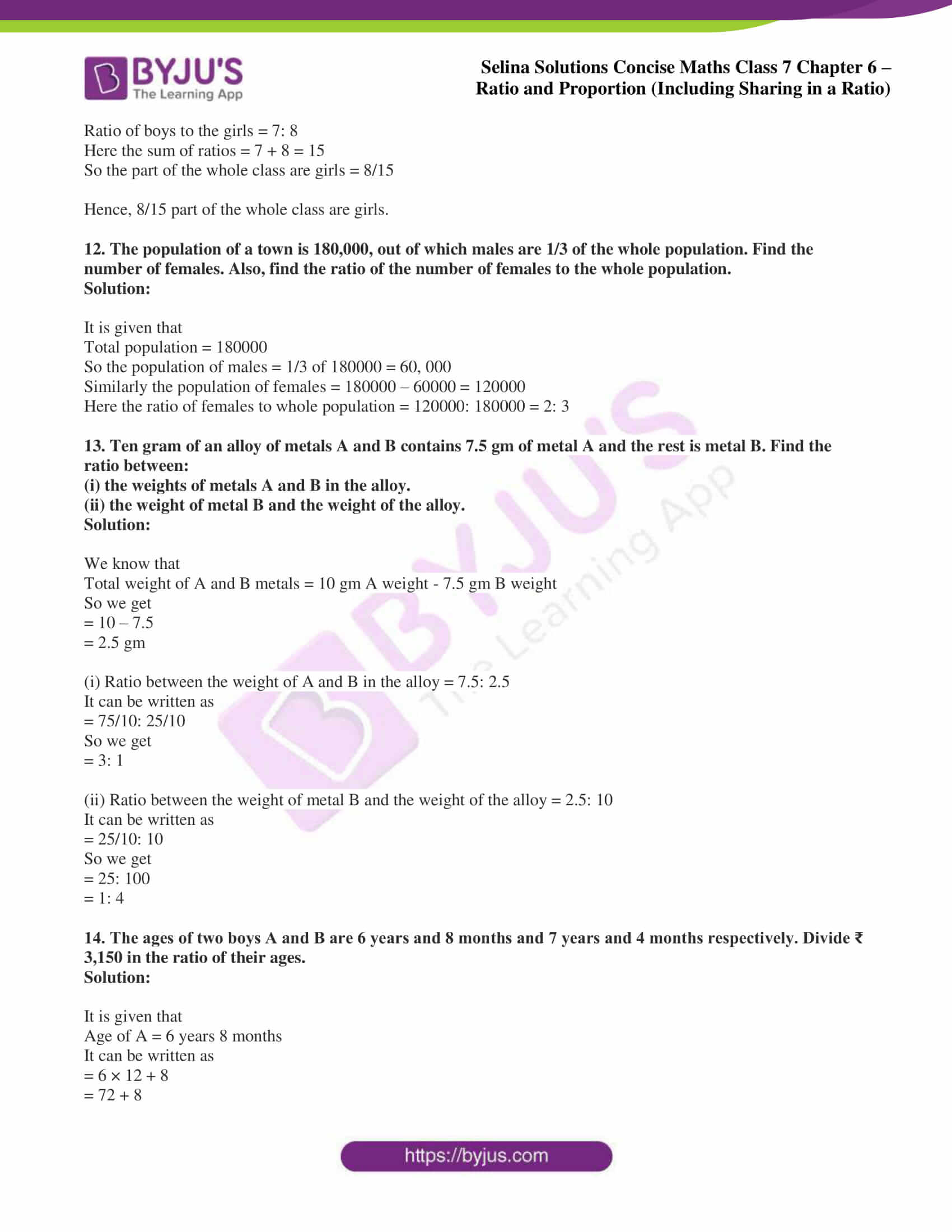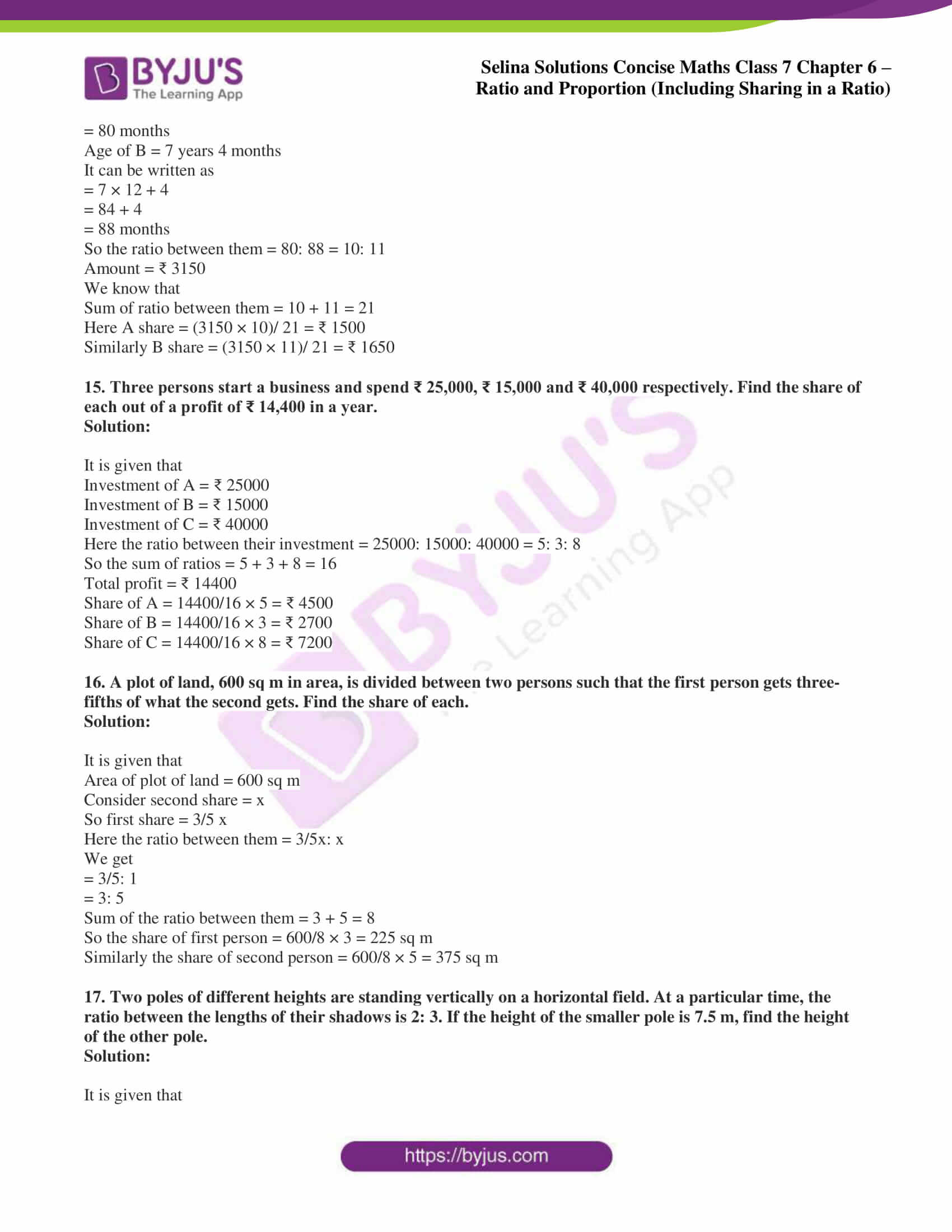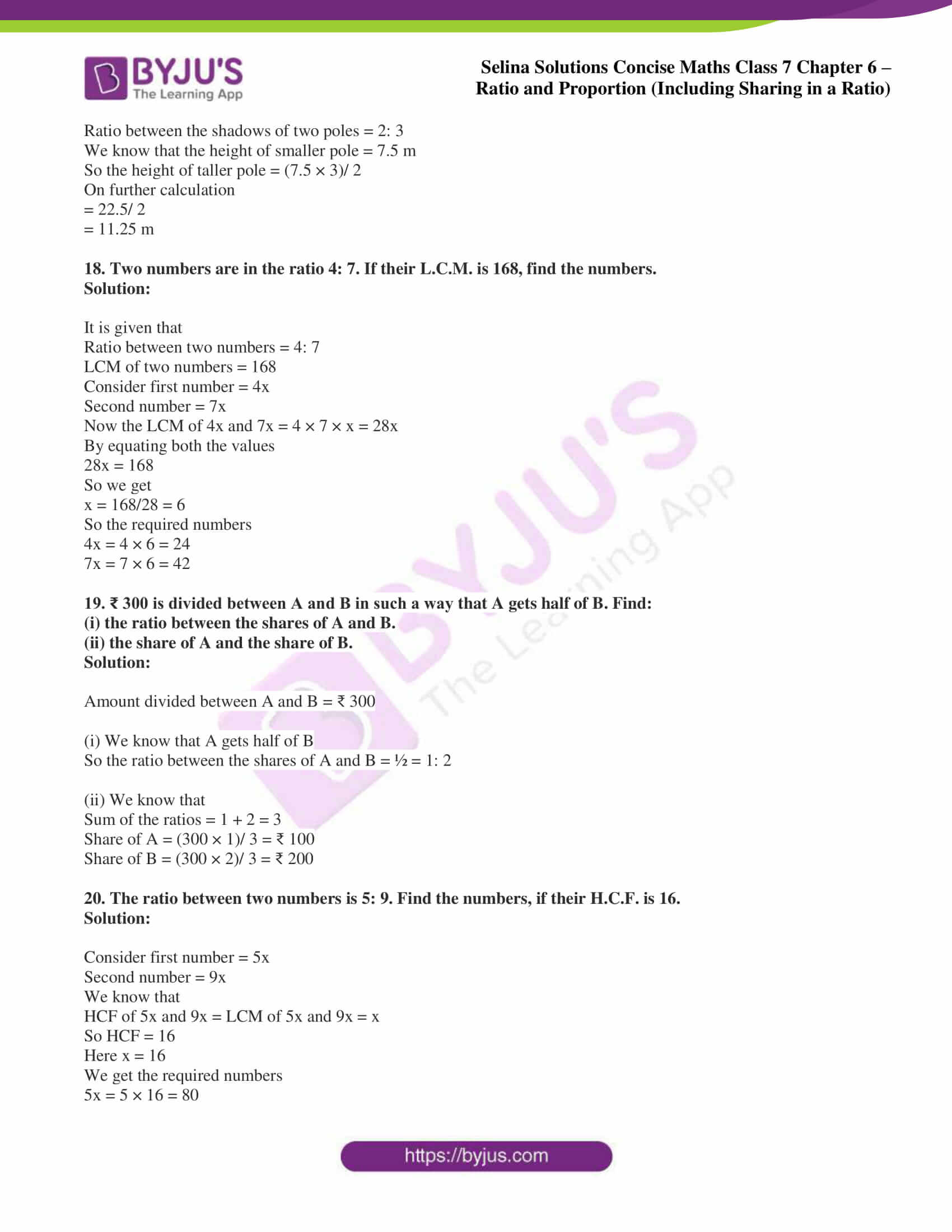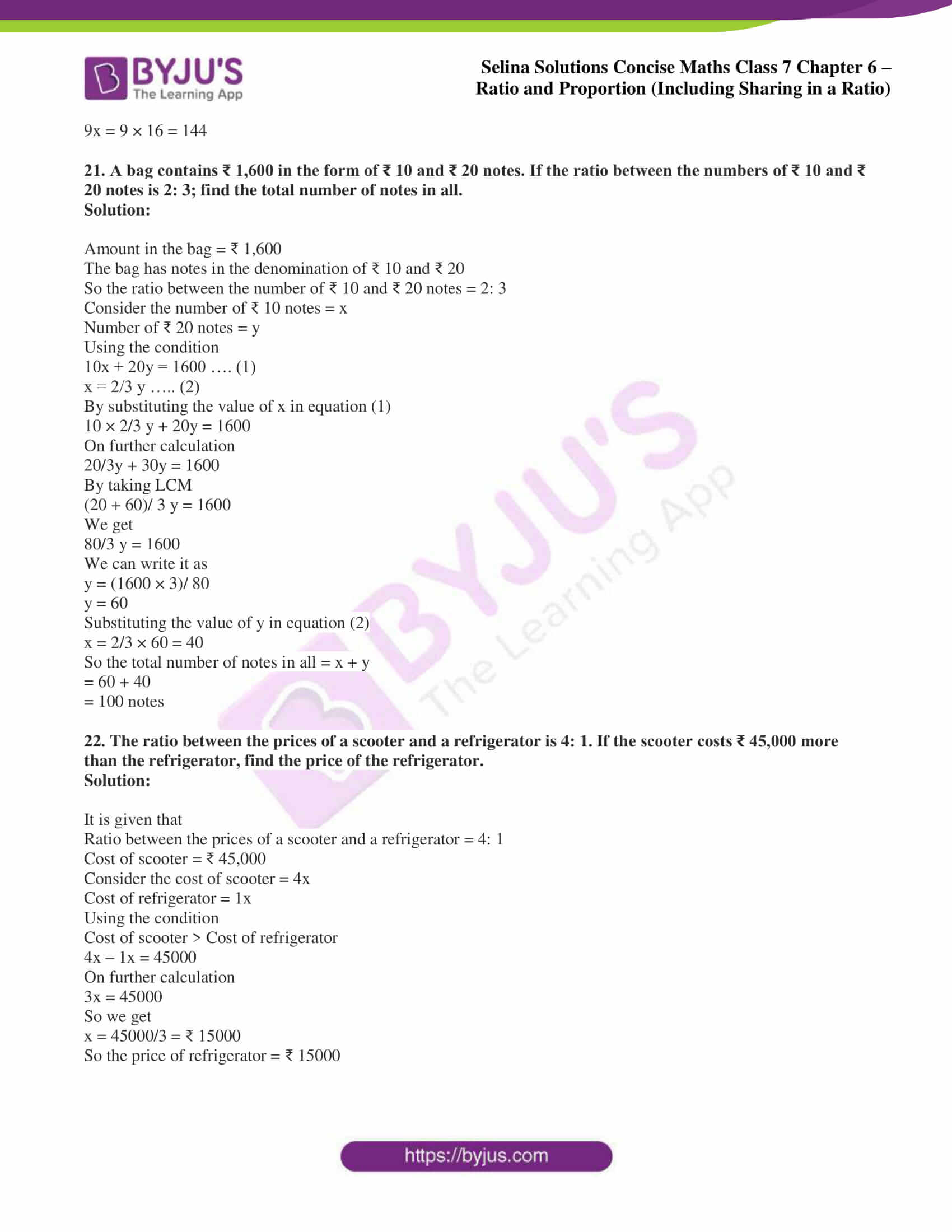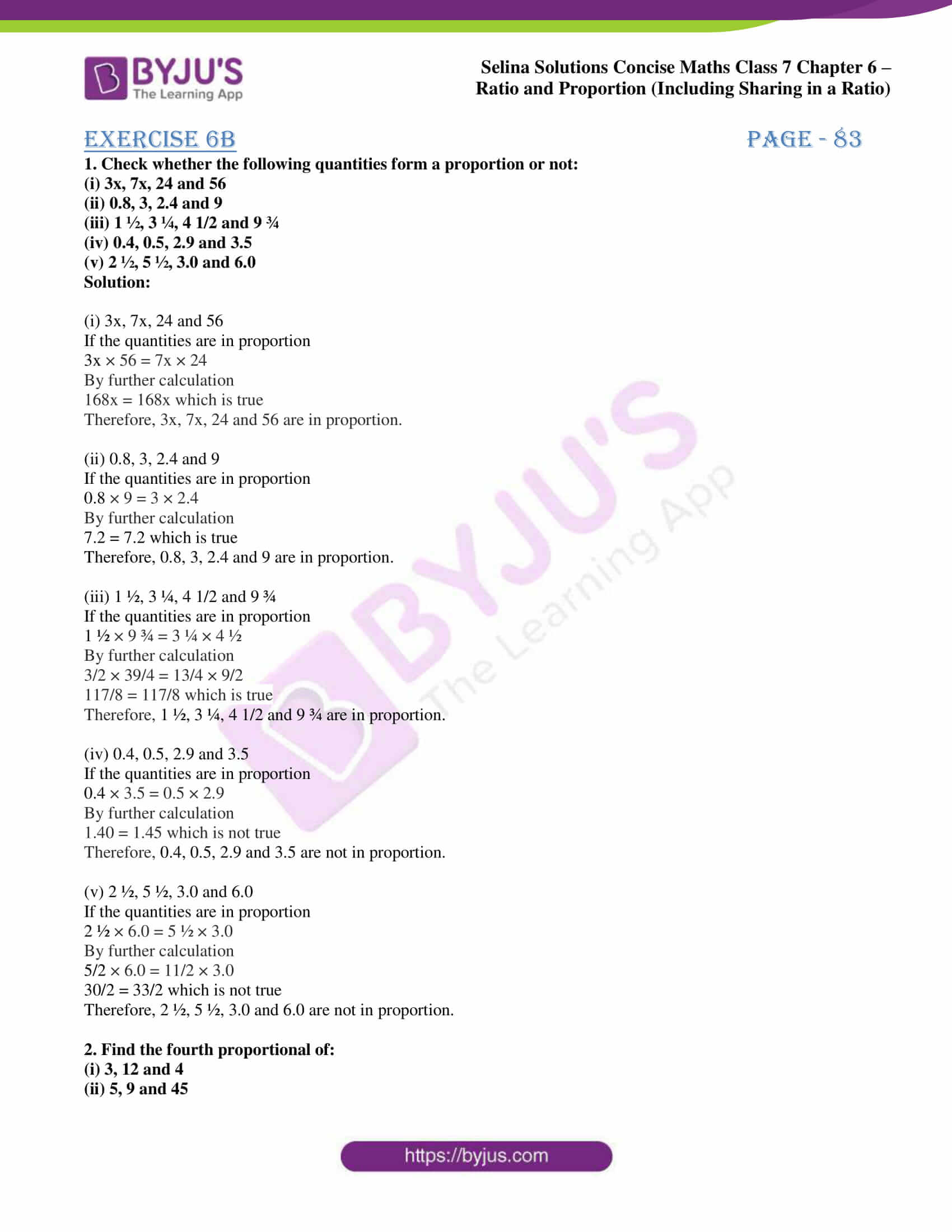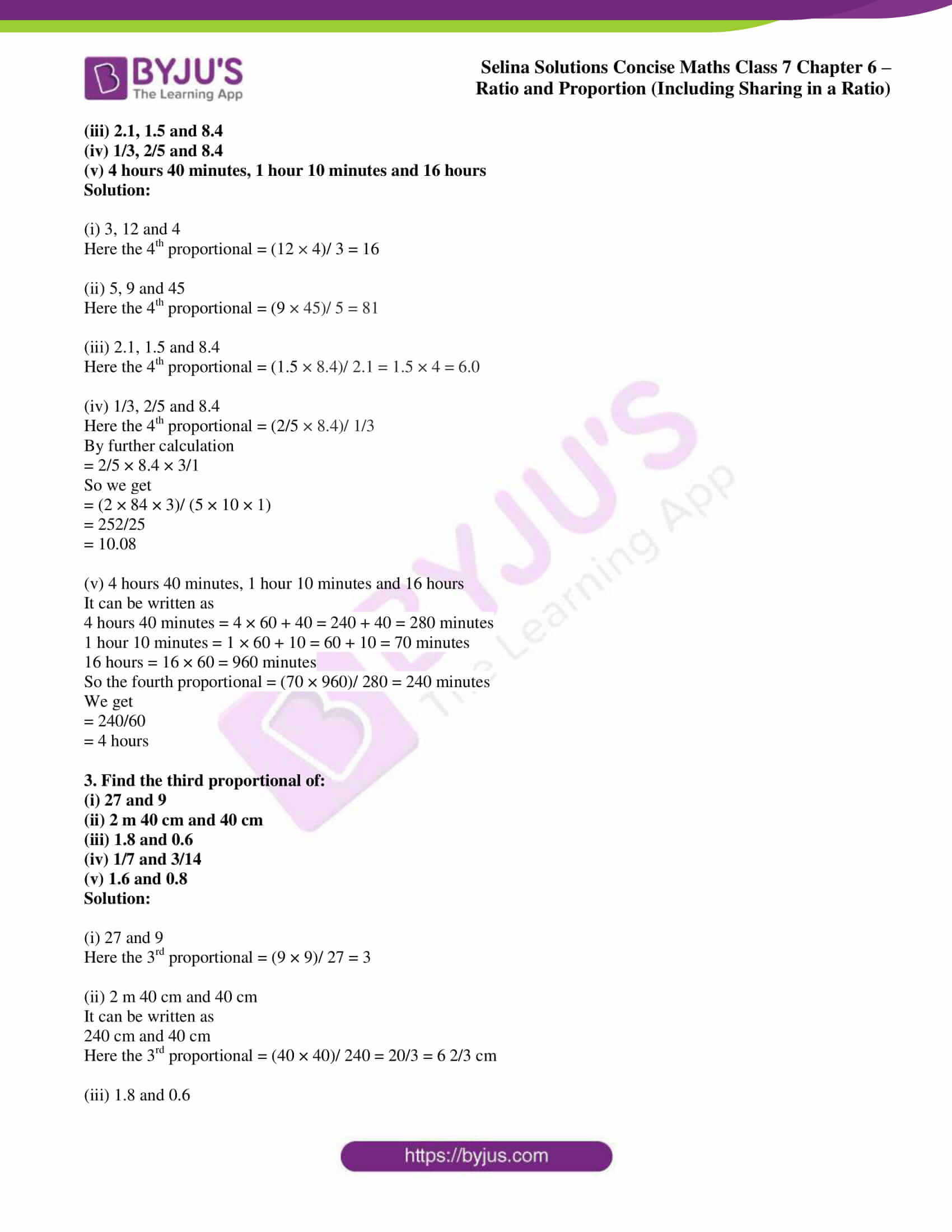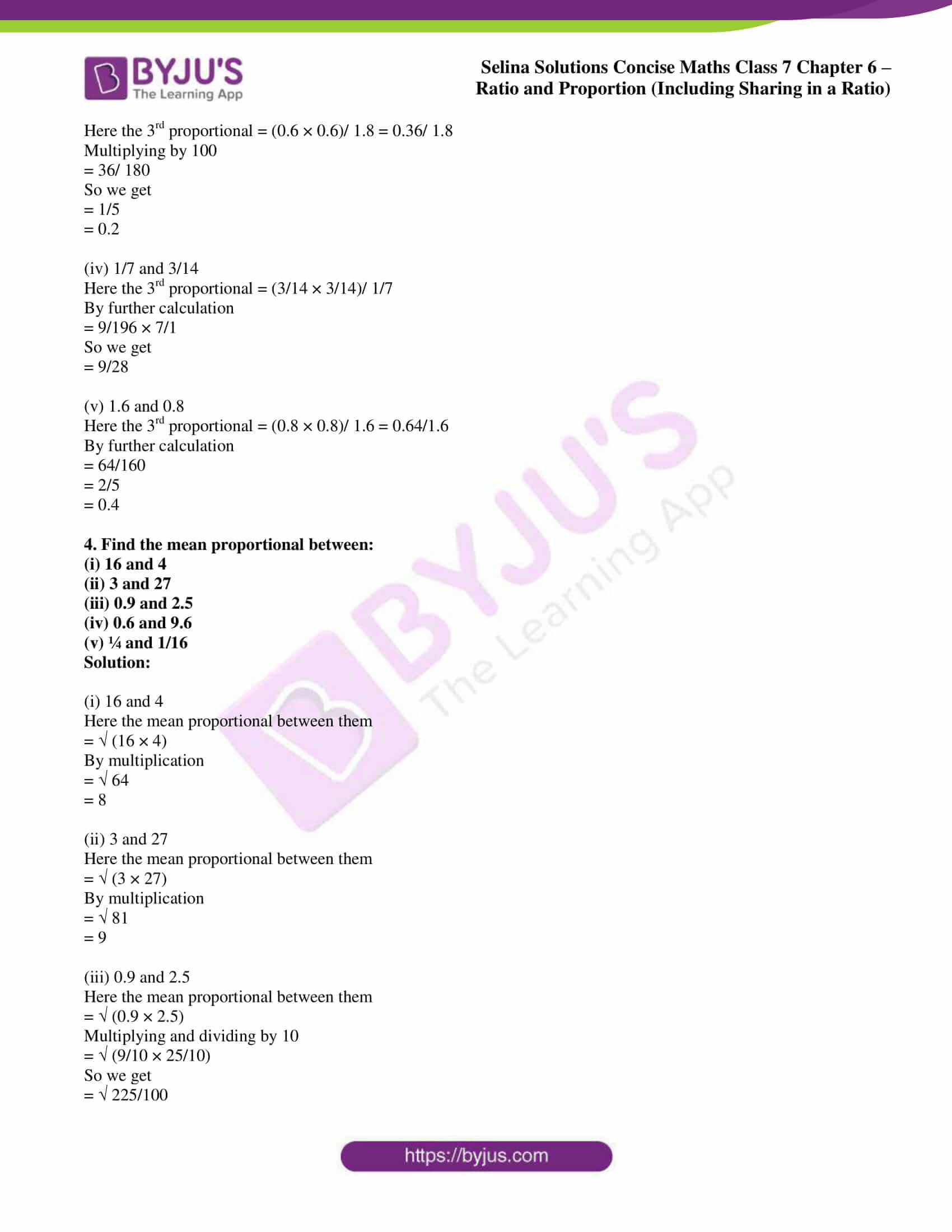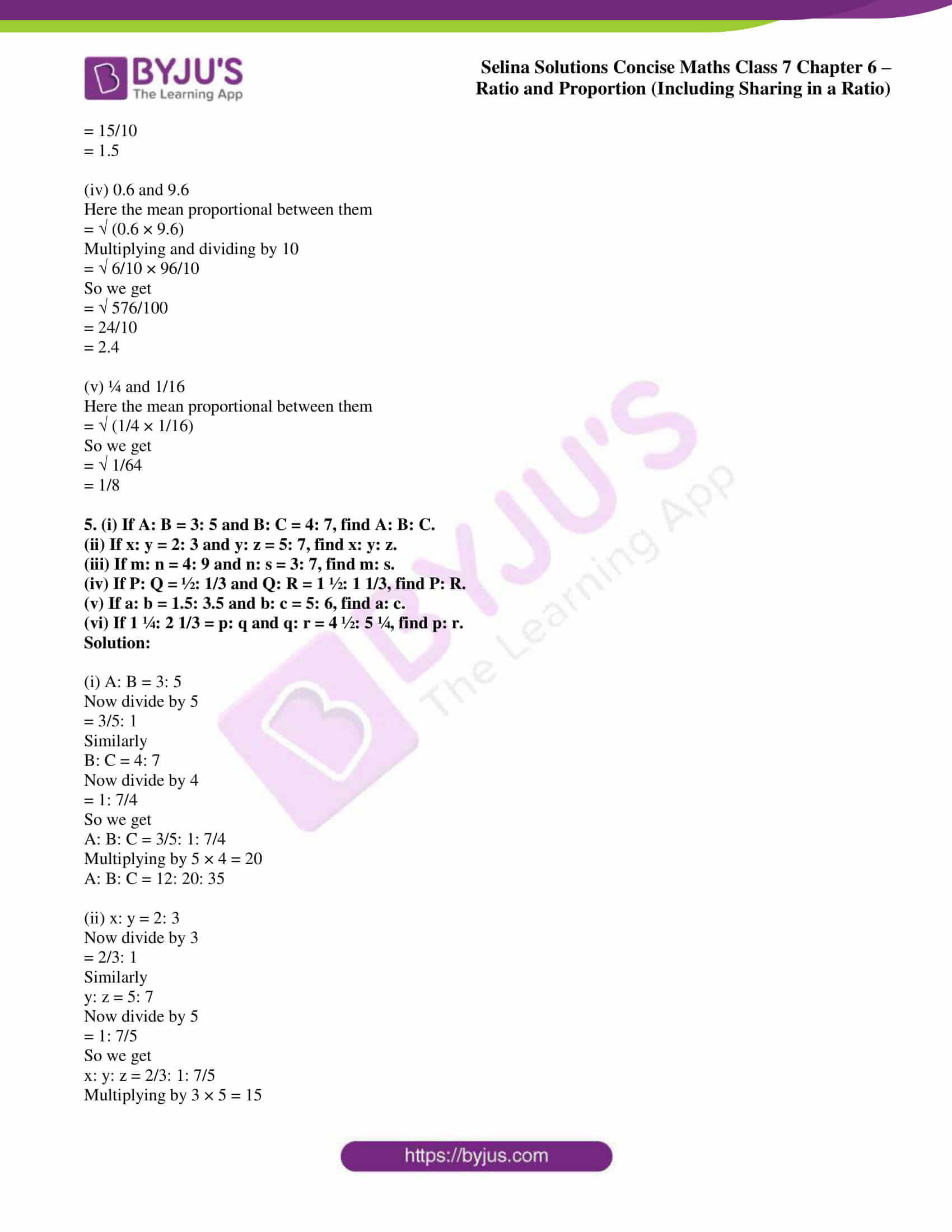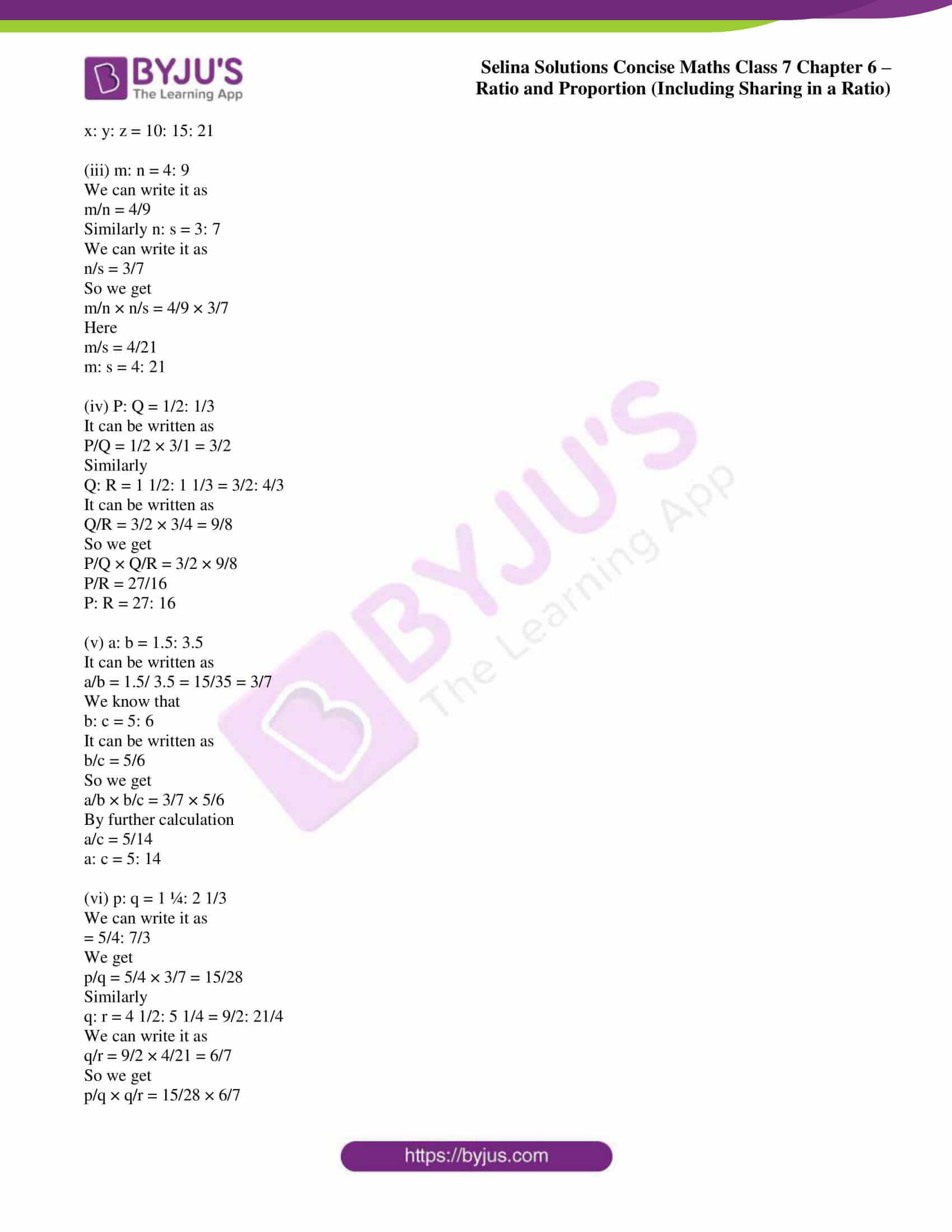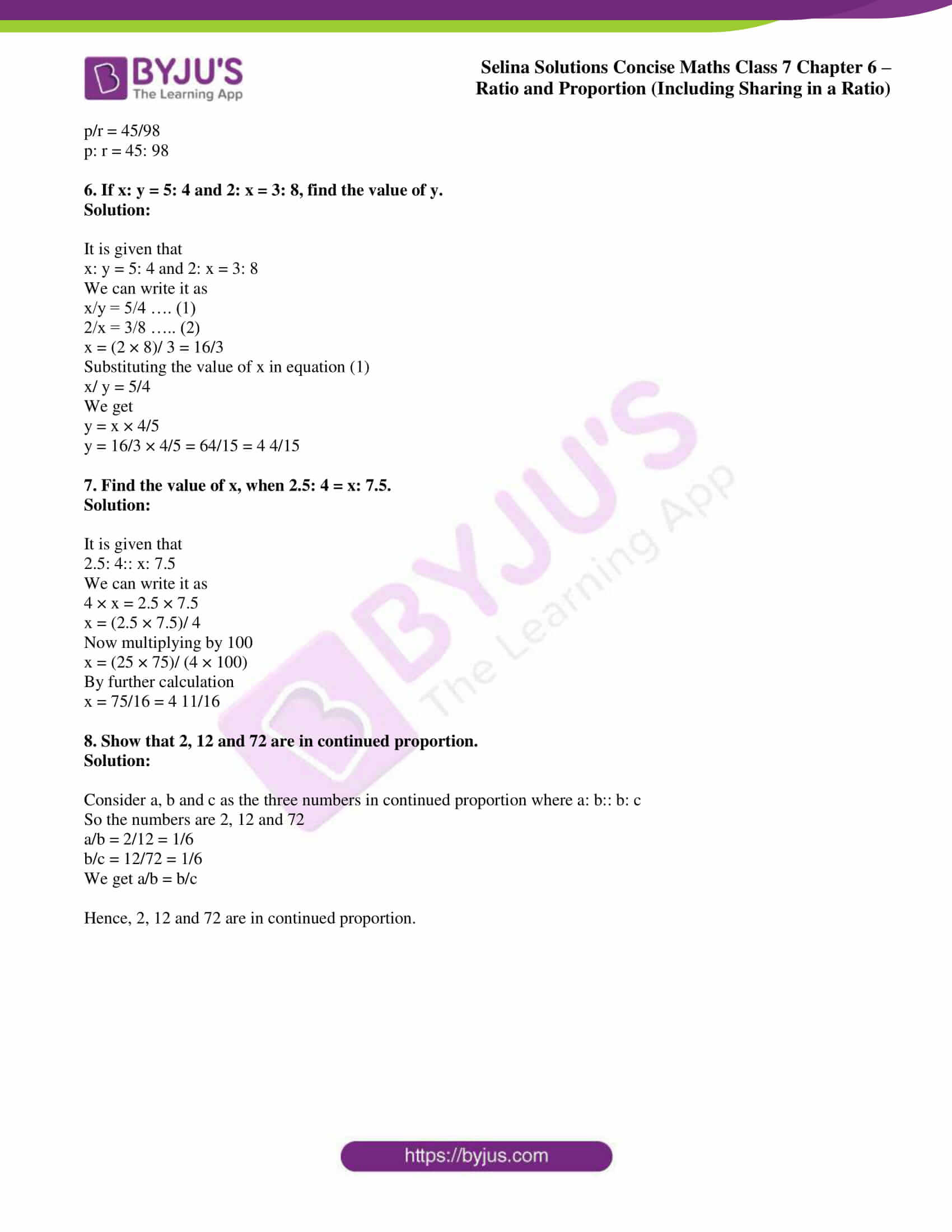## Access Selina Solutions Concise Maths Class 7 Chapter 6: Ratio and Proportion (Including Sharing in a Ratio)

#### Exercise 6A page – 80

1. Express each of the given ratios in its simplest form:

(i) 22: 66

(ii) 1.5: 2.5

(iii) 6 1/4: 12 1/2

(iv) 40 kg: 1 quintal

(v) 10 paise: ₹ 1

(vi) 200 m: 5 km

(vii) 3 hours: 1 day

(viii) 6 months: 1 1/3 years

(ix) 1 1/3: 2 1/4: 2 1/2

Solution:

(i) 22: 66

It can be written as

= 22/66

We know that the HCF of 22 and 66 is 22

Dividing both numerator and denominator by 22

= (22 ÷ 22)/ (66 ÷ 22)

So we get

= 1/3

= 1: 3

(ii) 1.5: 2.5

It can be written as

= 1.5/ 2.5

Multiplying both numerator and denominator by 10

= 15/25

We know that the HCF of 15 and 25 is 5

Dividing both numerator and denominator by 5

= (15 ÷ 5)/ (25 ÷ 5)

So we get

= 3/5

= 3: 5

(iii) 6 1/4: 12 1/2

It can be written as

= 25/4: 25/2

= 25/4 × 2/25

By further calculation

= 2/4

So we get

= 1/2

= 1: 2

(iv) 40 kg: 1 quintal

We know that

1 quintal = 100 kg

We get

= 40 kg: 100 kg

It can be written as

= 40/100

We know that the HCF of 40 and 100 is 20

Dividing both numerator and denominator by 20

= (40 ÷ 20)/ (100 ÷ 20)

So we get

= 2/5

= 2: 5

(v) 10 paise: ₹ 1

We know that

1 Rupee = 100 Paise

We get

= 10 paise: 100 paise

It can be written as

= 10/100

So we get

= 1/10

= 1: 10

(vi) 200 m: 5 km

We know that

1 km = 1000 m

We get

= 200 m: 5000 m

It can be written as

= 200/ 5000

Here the HCF of 200 and 5000 is 200

Dividing both numerator and denominator by 200

= (200 ÷ 200)/ (5000 ÷ 200)

So we get

= 1/25

= 1: 25

(vii) 3 hours: 1 day

We know that

1 day = 24 hours

We get

= 3 hours: 24 hours

It can be written as

= 3/24

So we get

= 1/ 8

= 1: 8

(viii) 6 months: 1 1/3 years

We know that

1 year = 12 months

We get

= 6 months: 4/3 × 12 months

It can be written as

= 6 months: 16 months

= 6/ 16

Here the HCF of 6 and 16 is 2

Dividing both numerator and denominator by 2

= (6 ÷ 2)/ (16 ÷ 2)

So we get

= 3/ 8

= 3: 8

(ix) 1 1/3: 2 1/4: 2 1/2

It can be written as

= 4/3: 9/4: 5/2

We know that the LCM of 3, 4 and 2 is 12

= (16: 27: 30)/ 12

So we get

= 16: 27: 30

2. Divide 64 cm long string into two parts in the ratio 5: 3.

Solution:

We know that

The sum of ratios = 5 + 3 = 8

So the first part = 5/8 of 64 cm = 40 cm

Similarly the second part = 3/8 of 64 cm = 24 cm

3. ₹ 720 is divided between x and y in the ratio 4: 5. How many rupees will each get?

Solution:

It is given that

Total amount = ₹ 720

Ratio between x and y = 4: 5

We know that

The sum of ratios = 4 + 5 = 9

So x’s share = 4/9 of ₹ 720 = ₹ 320

Similarly y’s share = 5/9 of ₹ 720 = ₹ 400

4. The angles of a triangle are in the ratio 3: 2: 7. Find each angle.

Solution:

It is given that

Ratios in angles of a triangle = 3: 2: 7

We know that

The sum of ratios = 3 + 2 + 7 = 12

In a triangle, the sum of all the angles = 180o

So the first angle of the triangle = 3/12 × 180o = 45o

Second angle of the triangle = 2/12 × 180o = 30o

Similarly the third angle of the triangle = 7/12 × 180o = 105o

5. A rectangular field is 100 m by 80 m. Find the ratio of:

Solution:

It is given that

Length of the rectangular field = 100 m

Breadth of the rectangular field = 80 m

So the perimeter = 2 (length + breadth)

= 2 (100 + 80) m

By further calculation

= 2 × 180

= 360 m

(i) Ratio of length to its breadth

= 100: 80

Here the HCF of 100 and 80 is 20

Dividing both numerator and denominator by 20

= (100 ÷ 20)/ (80 ÷ 20)

So we get

= 5/4

= 5: 4

(ii) Ratio of breadth to its perimeter

= 80: 360

Here the HCF of 80 and 360 is 40

Dividing both numerator and denominator by 40

= (80 ÷ 40)/ (360 ÷ 40)

So we get

= 2/9

= 2: 9

6. The sum of three numbers, whose ratios are 3 1/3: 4 1/5: 6 1/8 is 4917. Find the numbers.

Solution:

It is given that

Sum of three numbers = 4917

Ratio between the three numbers = 3 1/3: 4 1/5: 6 1/8

It can be written as

= 10/3: 21/5: 49/8

We know that the LCM of 3, 5 and 8 is 120

= (400: 504: 735)/ 120

So we get

= 400: 504: 735

Here the sum of ratio = 400 + 504 + 735 = 1639

So the first number = 400/1639 of 4917 = 1200

Second number = 504/1639 of 4917 = 1512

Similarly the third number = 735/1639 of 4917 = 2205

7. The ratio between two quantities is 3: 4. If the first is ₹ 810, find the second.

Solution:

It is given that

The ratio between two quantities = 3: 4

So the sum of ratio = 3 + 4 = 7

Here the second quantity = (810 × 4)/ 3

We get

= 270 × 4

= ₹ 1080

8. Two numbers are in the ratio 5: 7. Their difference is 10. Find the numbers.

Solution:

It is given that

The ratio between two numbers = 5: 7

The difference between two numbers = 7 – 5 = 2

Here if 2 is the difference, the first number is 5

Similarly if 10 if the difference, the first number = 5/2 × 10 = 25

Second number = 7/2 × 10 = 35

9. Two numbers are in the ratio 10: 11. Their sum is 168. Find the numbers.

Solution:

It is given that

The ratio between two numbers = 10: 11

Sum of ratio between two numbers = 10 + 11 = 21

Sum of two numbers = 168

So the first number = 168/21 × 10 = 80

Similarly the second number = 168/21 × 11 = 88

10. A line is divided into two parts in the ratio 2.5: 1.3. If the smaller one is 35.1 cm, find the length of the line.

Solution:

It is given that

Ratio between two parts of a line = 2.5: 1.3

Multiplying by 10

= 25: 13

Here the sum of ratios = 25 + 13 = 38

Length of smaller one = 35.1 cm

So the length of the line = 38/13 × 35.1

We get

= 38 × 2.7 cm

= 102.6 cm

11. In a class, the ratio of boys to the girls is 7:8. What part of the whole class are girls?

Solution:

It is given that

Ratio of boys to the girls = 7: 8

Here the sum of ratios = 7 + 8 = 15

So the part of the whole class are girls = 8/15

Hence, 8/15 part of the whole class are girls.

12. The population of a town is 180,000, out of which males are 1/3 of the whole population. Find the number of females. Also, find the ratio of the number of females to the whole population.

Solution:

It is given that

Total population = 180000

So the population of males = 1/3 of 180000 = 60, 000

Similarly the population of females = 180000 – 60000 = 120000

Here the ratio of females to whole population = 120000: 180000 = 2: 3

13. Ten gram of an alloy of metals A and B contains 7.5 gm of metal A and the rest is metal B. Find the ratio between:

(i) the weights of metals A and B in the alloy.

(ii) the weight of metal B and the weight of the alloy.

Solution:

We know that

Total weight of A and B metals = 10 gm A weight – 7.5 gm B weight

So we get

= 10 – 7.5

= 2.5 gm

(i) Ratio between the weight of A and B in the alloy = 7.5: 2.5

It can be written as

= 75/10: 25/10

So we get

= 3: 1

(ii) Ratio between the weight of metal B and the weight of the alloy = 2.5: 10

It can be written as

= 25/10: 10

So we get

= 25: 100

= 1: 4

14. The ages of two boys A and B are 6 years and 8 months and 7 years and 4 months respectively. Divide ₹ 3,150 in the ratio of their ages.

Solution:

It is given that

Age of A = 6 years 8 months

It can be written as

= 6 × 12 + 8

= 72 + 8

= 80 months

Age of B = 7 years 4 months

It can be written as

= 7 × 12 + 4

= 84 + 4

= 88 months

So the ratio between them = 80: 88 = 10: 11

Amount = ₹ 3150

We know that

Sum of ratio between them = 10 + 11 = 21

Here A share = (3150 × 10)/ 21 = ₹ 1500

Similarly B share = (3150 × 11)/ 21 = ₹ 1650

15. Three persons start a business and spend ₹ 25,000, ₹ 15,000 and ₹ 40,000 respectively. Find the share of each out of a profit of ₹ 14,400 in a year.

Solution:

It is given that

Investment of A = ₹ 25000

Investment of B = ₹ 15000

Investment of C = ₹ 40000

Here the ratio between their investment = 25000: 15000: 40000 = 5: 3: 8

So the sum of ratios = 5 + 3 + 8 = 16

Total profit = ₹ 14400

Share of A = 14400/16 × 5 = ₹ 4500

Share of B = 14400/16 × 3 = ₹ 2700

Share of C = 14400/16 × 8 = ₹ 7200

16. A plot of land, 600 sq m in area, is divided between two persons such that the first person gets three-fifths of what the second gets. Find the share of each.

Solution:

It is given that

Area of plot of land = 600 sq m

Consider second share = x

So first share = 3/5 x

Here the ratio between them = 3/5x: x

We get

= 3/5: 1

= 3: 5

Sum of the ratio between them = 3 + 5 = 8

So the share of first person = 600/8 × 3 = 225 sq m

Similarly the share of second person = 600/8 × 5 = 375 sq m

17. Two poles of different heights are standing vertically on a horizontal field. At a particular time, the ratio between the lengths of their shadows is 2: 3. If the height of the smaller pole is 7.5 m, find the height of the other pole.

Solution:

It is given that

Ratio between the shadows of two poles = 2: 3

We know that the height of smaller pole = 7.5 m

So the height of taller pole = (7.5 × 3)/ 2

On further calculation

= 22.5/ 2

= 11.25 m

18. Two numbers are in the ratio 4: 7. If their L.C.M. is 168, find the numbers.

Solution:

It is given that

Ratio between two numbers = 4: 7

LCM of two numbers = 168

Consider first number = 4x

Second number = 7x

Now the LCM of 4x and 7x = 4 × 7 × x = 28x

By equating both the values

28x = 168

So we get

x = 168/28 = 6

So the required numbers

4x = 4 × 6 = 24

7x = 7 × 6 = 42

19. ₹ 300 is divided between A and B in such a way that A gets half of B. Find:

(i) the ratio between the shares of A and B.

(ii) the share of A and the share of B.

Solution:

Amount divided between A and B = ₹ 300

(i) We know that A gets half of B

So the ratio between the shares of A and B = ½ = 1: 2

(ii) We know that

Sum of the ratios = 1 + 2 = 3

Share of A = (300 × 1)/ 3 = ₹ 100

Share of B = (300 × 2)/ 3 = ₹ 200

20. The ratio between two numbers is 5: 9. Find the numbers, if their H.C.F. is 16.

Solution:

Consider first number = 5x

Second number = 9x

We know that

HCF of 5x and 9x = LCM of 5x and 9x = x

So HCF = 16

Here x = 16

We get the required numbers

5x = 5 × 16 = 80

9x = 9 × 16 = 144

21. A bag contains ₹ 1,600 in the form of ₹ 10 and ₹ 20 notes. If the ratio between the numbers of ₹ 10 and ₹ 20 notes is 2: 3; find the total number of notes in all.

Solution:

Amount in the bag = ₹ 1,600

The bag has notes in the denomination of ₹ 10 and ₹ 20

So the ratio between the number of ₹ 10 and ₹ 20 notes = 2: 3

Consider the number of ₹ 10 notes = x

Number of ₹ 20 notes = y

Using the condition

10x + 20y = 1600 …. (1)

x = 2/3 y ….. (2)

By substituting the value of x in equation (1)

10 × 2/3 y + 20y = 1600

On further calculation

20/3y + 30y = 1600

By taking LCM

(20 + 60)/ 3 y = 1600

We get

80/3 y = 1600

We can write it as

y = (1600 × 3)/ 80

y = 60

Substituting the value of y in equation (2)

x = 2/3 × 60 = 40

So the total number of notes in all = x + y

= 60 + 40

= 100 notes

22. The ratio between the prices of a scooter and a refrigerator is 4: 1. If the scooter costs ₹ 45,000 more than the refrigerator, find the price of the refrigerator.

Solution:

It is given that

Ratio between the prices of a scooter and a refrigerator = 4: 1

Cost of scooter = ₹ 45,000

Consider the cost of scooter = 4x

Cost of refrigerator = 1x

Using the condition

Cost of scooter > Cost of refrigerator

4x – 1x = 45000

On further calculation

3x = 45000

So we get

x = 45000/3 = ₹ 15000

So the price of refrigerator = ₹ 15000

#### Exercise 6B page – 83

1. Check whether the following quantities form a proportion or not:

(i) 3x, 7x, 24 and 56

(ii) 0.8, 3, 2.4 and 9

(iii) 1 ½, 3 ¼, 4 1/2 and 9 ¾

(iv) 0.4, 0.5, 2.9 and 3.5

(v) 2 ½, 5 ½, 3.0 and 6.0

Solution:

(i) 3x, 7x, 24 and 56

If the quantities are in proportion

3x × 56 = 7x × 24

By further calculation

168x = 168x which is true

Therefore, 3x, 7x, 24 and 56 are in proportion.

(ii) 0.8, 3, 2.4 and 9

If the quantities are in proportion

0.8 × 9 = 3 × 2.4

By further calculation

7.2 = 7.2 which is true

Therefore, 0.8, 3, 2.4 and 9 are in proportion.

(iii) 1 ½, 3 ¼, 4 1/2 and 9 ¾

If the quantities are in proportion

1 ½ × 9 ¾ = 3 ¼ × 4 ½

By further calculation

3/2 × 39/4 = 13/4 × 9/2

117/8 = 117/8 which is true

Therefore, 1 ½, 3 ¼, 4 1/2 and 9 ¾ are in proportion.

(iv) 0.4, 0.5, 2.9 and 3.5

If the quantities are in proportion

0.4 × 3.5 = 0.5 × 2.9

By further calculation

1.40 = 1.45 which is not true

Therefore, 0.4, 0.5, 2.9 and 3.5 are not in proportion.

(v) 2 ½, 5 ½, 3.0 and 6.0

If the quantities are in proportion

2 ½ × 6.0 = 5 ½ × 3.0

By further calculation

5/2 × 6.0 = 11/2 × 3.0

30/2 = 33/2 which is not true

Therefore, 2 ½, 5 ½, 3.0 and 6.0 are not in proportion.

2. Find the fourth proportional of:

(i) 3, 12 and 4

(ii) 5, 9 and 45

(iii) 2.1, 1.5 and 8.4

(iv) 1/3, 2/5 and 8.4

(v) 4 hours 40 minutes, 1 hour 10 minutes and 16 hours

Solution:

(i) 3, 12 and 4

Here the 4th proportional = (12 × 4)/ 3 = 16

(ii) 5, 9 and 45

Here the 4th proportional = (9 × 45)/ 5 = 81

(iii) 2.1, 1.5 and 8.4

Here the 4th proportional = (1.5 × 8.4)/ 2.1 = 1.5 × 4 = 6.0

(iv) 1/3, 2/5 and 8.4

Here the 4th proportional = (2/5 × 8.4)/ 1/3

By further calculation

= 2/5 × 8.4 × 3/1

So we get

= (2 × 84 × 3)/ (5 × 10 × 1)

= 252/25

= 10.08

(v) 4 hours 40 minutes, 1 hour 10 minutes and 16 hours

It can be written as

4 hours 40 minutes = 4 × 60 + 40 = 240 + 40 = 280 minutes

1 hour 10 minutes = 1 × 60 + 10 = 60 + 10 = 70 minutes

16 hours = 16 × 60 = 960 minutes

So the fourth proportional = (70 × 960)/ 280 = 240 minutes

We get

= 240/60

= 4 hours

3. Find the third proportional of:

(i) 27 and 9

(ii) 2 m 40 cm and 40 cm

(iii) 1.8 and 0.6

(iv) 1/7 and 3/14

(v) 1.6 and 0.8

Solution:

(i) 27 and 9

Here the 3rd proportional = (9 × 9)/ 27 = 3

(ii) 2 m 40 cm and 40 cm

It can be written as

240 cm and 40 cm

Here the 3rd proportional = (40 × 40)/ 240 = 20/3 = 6 2/3 cm

(iii) 1.8 and 0.6

Here the 3rd proportional = (0.6 × 0.6)/ 1.8 = 0.36/ 1.8

Multiplying by 100

= 36/ 180

So we get

= 1/5

= 0.2

(iv) 1/7 and 3/14

Here the 3rd proportional = (3/14 × 3/14)/ 1/7

By further calculation

= 9/196 × 7/1

So we get

= 9/28

(v) 1.6 and 0.8

Here the 3rd proportional = (0.8 × 0.8)/ 1.6 = 0.64/1.6

By further calculation

= 64/160

= 2/5

= 0.4

4. Find the mean proportional between:

(i) 16 and 4

(ii) 3 and 27

(iii) 0.9 and 2.5

(iv) 0.6 and 9.6

(v) ¼ and 1/16

Solution:

(i) 16 and 4

Here the mean proportional between them

= √ (16 × 4)

By multiplication

= √ 64

= 8

(ii) 3 and 27

Here the mean proportional between them

= √ (3 × 27)

By multiplication

= √ 81

= 9

(iii) 0.9 and 2.5

Here the mean proportional between them

= √ (0.9 × 2.5)

Multiplying and dividing by 10

= √ (9/10 × 25/10)

So we get

= √ 225/100

= 15/10

= 1.5

(iv) 0.6 and 9.6

Here the mean proportional between them

= √ (0.6 × 9.6)

Multiplying and dividing by 10

= √ 6/10 × 96/10

So we get

= √ 576/100

= 24/10

= 2.4

(v) ¼ and 1/16

Here the mean proportional between them

= √ (1/4 × 1/16)

So we get

= √ 1/64

= 1/8

5. (i) If A: B = 3: 5 and B: C = 4: 7, find A: B: C.

(ii) If x: y = 2: 3 and y: z = 5: 7, find x: y: z.

(iii) If m: n = 4: 9 and n: s = 3: 7, find m: s.

(iv) If P: Q = ½: 1/3 and Q: R = 1 ½: 1 1/3, find P: R.

(v) If a: b = 1.5: 3.5 and b: c = 5: 6, find a: c.

(vi) If 1 ¼: 2 1/3 = p: q and q: r = 4 ½: 5 ¼, find p: r.

Solution:

(i) A: B = 3: 5

Now divide by 5

= 3/5: 1

Similarly

B: C = 4: 7

Now divide by 4

= 1: 7/4

So we get

A: B: C = 3/5: 1: 7/4

Multiplying by 5 × 4 = 20

A: B: C = 12: 20: 35

(ii) x: y = 2: 3

Now divide by 3

= 2/3: 1

Similarly

y: z = 5: 7

Now divide by 5

= 1: 7/5

So we get

x: y: z = 2/3: 1: 7/5

Multiplying by 3 × 5 = 15

x: y: z = 10: 15: 21

(iii) m: n = 4: 9

We can write it as

m/n = 4/9

Similarly n: s = 3: 7

We can write it as

n/s = 3/7

So we get

m/n × n/s = 4/9 × 3/7

Here

m/s = 4/21

m: s = 4: 21

(iv) P: Q = 1/2: 1/3

It can be written as

P/Q = 1/2 × 3/1 = 3/2

Similarly

Q: R = 1 1/2: 1 1/3 = 3/2: 4/3

It can be written as

Q/R = 3/2 × 3/4 = 9/8

So we get

P/Q × Q/R = 3/2 × 9/8

P/R = 27/16

P: R = 27: 16

(v) a: b = 1.5: 3.5

It can be written as

a/b = 1.5/ 3.5 = 15/35 = 3/7

We know that

b: c = 5: 6

It can be written as

b/c = 5/6

So we get

a/b × b/c = 3/7 × 5/6

By further calculation

a/c = 5/14

a: c = 5: 14

(vi) p: q = 1 ¼: 2 1/3

We can write it as

= 5/4: 7/3

We get

p/q = 5/4 × 3/7 = 15/28

Similarly

q: r = 4 1/2: 5 1/4 = 9/2: 21/4

We can write it as

q/r = 9/2 × 4/21 = 6/7

So we get

p/q × q/r = 15/28 × 6/7

p/r = 45/98

p: r = 45: 98

6. If x: y = 5: 4 and 2: x = 3: 8, find the value of y.

Solution:

It is given that

x: y = 5: 4 and 2: x = 3: 8

We can write it as

x/y = 5/4 …. (1)

2/x = 3/8 ….. (2)

x = (2 × 8)/ 3 = 16/3

Substituting the value of x in equation (1)

x/ y = 5/4

We get

y = x × 4/5

y = 16/3 × 4/5 = 64/15 = 4 4/15

7. Find the value of x, when 2.5: 4 = x: 7.5.

Solution:

It is given that

2.5: 4:: x: 7.5

We can write it as

4 × x = 2.5 × 7.5

x = (2.5 × 7.5)/ 4

Now multiplying by 100

x = (25 × 75)/ (4 × 100)

By further calculation

x = 75/16 = 4 11/16

8. Show that 2, 12 and 72 are in continued proportion.

Solution:

Consider a, b and c as the three numbers in continued proportion where a: b:: b: c

So the numbers are 2, 12 and 72

a/b = 2/12 = 1/6

b/c = 12/72 = 1/6

We get a/b = b/c

Hence, 2, 12 and 72 are in continued proportion.